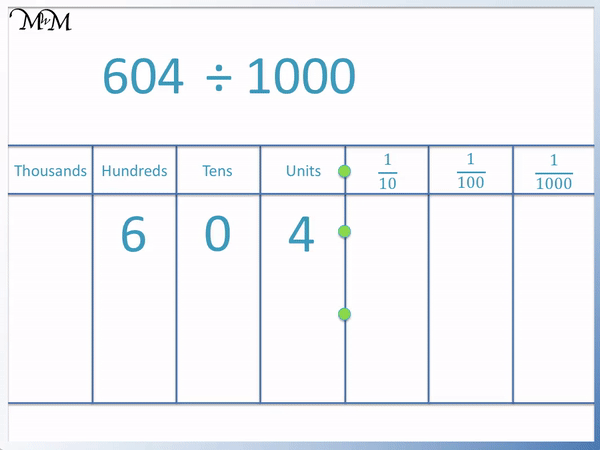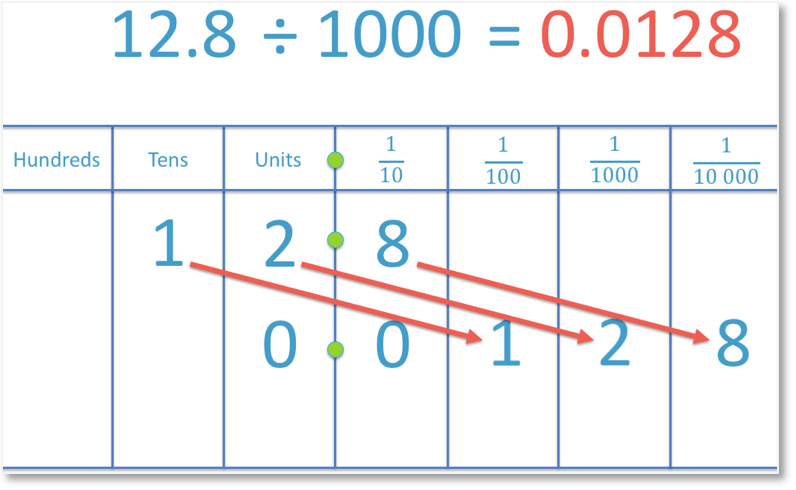# Dividing by 1000

Dividing by 1000• To divide a number by 1000, move all of its digits three place value columns to right.
• The digit ‘9’ will move from the thousands column to the units column.
• The digit ‘0’ will move from the hundreds column to the tenths column, immediately after the decimal point.
• The digit ‘0’ in the tens column will move to the hundredths column.
• The digit ‘0’ in the units column will move into the thousandths column
• 9000 ÷ 1000 = 9.000
• Since the digits after the decimal point are all zeros, we do not write them.
• 9000 ÷ 1000 = 9.
• Because 9000 is a whole number, ending in three ‘0’ digits, dividing it by 1000 had the same effect as removing these three zeros.
To divide by 1000, move all digits in a number 3 place value columns to the right.• To divide a number by 1000, move all of its digits 3 place value columns to the right.
• In this example we have 604 ÷ 1000.
• The ‘6’ in the hundreds column moves to the tenths column, immediately after the decimal point.
• The ‘0’ in the tens column moves to the hundredths column.
• The ‘4’ in the units column moves to the thousandths column.
• 604 ÷ 1000 = 0.604Supporting Lessons#### Dividing by 1000: Interactive Questions

Divide Decimals by 1000: Interactive Questions

# How to Divide a Number by 1000

To divide a number by 1000 we move each digit in that number three place value columns to the right.

Dividing a whole number that ends in three zeros by 1000 has the same effect as removing the three zeros.

This trick only works for whole numbers that end in three zeros (or numbers that are multiples of 1000). Here is an example of dividing such a number by 1000.In the example above, each digit in the number 9000 has been moved three places to the right.

The digit of ‘9’ in the thousands column moves into the units column.

When dividing by one thousand, any digit in the thousands column will always move into the units column to the left of the decimal point.

The ‘0’ in the hundreds column moves three places into the tenths column, immediately after the decimal point.

The ‘0’ in the tens column moves three places into the hundredths column.

Finally, the ‘0’ in the units column moves into the thousandths column.

So, 9000 becomes 9.000, which is the same as 9.

If a number only has the digit of ‘0’ after its decimal point, then it is a whole number and we do not write the zeros or the decimal point.

It is easier to write 9 than 9.000.

You may also know that when we divide a whole number ending in three zeros by 1000, that we can simply “remove the zeros” from the end of this number.

This trick will not work if we have a decimal number.

It is important to understand how dividing by 1000 works because not all numbers that we divide by 1000 will end in three zeros.

For example:To divide 604 by 1000, we move each digit in 604 three places to the right.

We begin by moving the ‘6’ from the hundreds column three places to the right into the tenths column.

The ‘0’ and the ‘4’ will then follow the 6 in the same order. The ‘0’ moves from the tens column into the hundredths column and the ‘4’ moves from the units column into the thousandths column.

Because there are no longer any digits in the units column, we write in a zero.

And so, 604 ÷ 1000 = 0.604.

We always write a single ‘0’ digit in the units column before the decimal point if there is no other digit remaining to write there. It is not correct to start a number with a decimal point and so we write ‘0.604’ rather than ‘.604’. This avoids confusion in written text involving numbers. It avoids confusion between commas, full stops and decimal points.

A decimal point is small and sometimes not noticed. By including the zero before the decimal point, it helps to identify to the reader that there is likely to be a decimal point after the zero. This is because whole numbers do not start with a zero and the only way for a zero to be the first digit in a number is if it is a decimal number.

In the following example we are dividing a decimal number by 1000.We begin by moving the digit of ‘1’ three places to the right. It moves from the tens column to the hundredths column. The 2 and the 8 will then follow. We move the 2 from the units column to the thousandths column and we move the 8 from the tenths column to the ten-thousandths column.

As there are no longer any digits in either the units column or the tenths column, we write a zero in each of these spaces.

Therefore, 12.8 ÷ 1000 = 0.0128

In the following example we are dividing a decimal number that is less than 1 by 1000.To do this, we move the digit of ‘5’ three places to the right, from the tenths column to the ten-thousandths column.

Because there are no digits in either the tenths column, the hundredths column or the thousandths column, we write a zero in each of these columns to show that their values are zero.

Therefore, 0.5 ÷ 1000 = 0.0005.Now try our lesson on Tenths and Hundredths where we understand more about the decimal place value columns of tenths and hundredths.